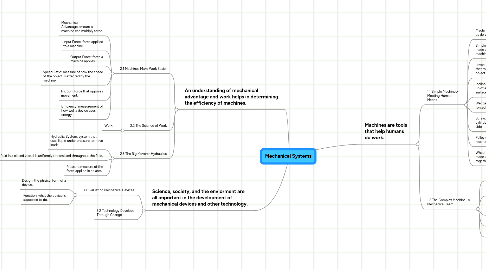# Mechanical Systems

Get Started. It's FreeMechanical Systems## 1. Machines are tools that help humans do work.

### 1.1. 1.1 Simple Machines- Meeting Human Needs

1.1.1. Machine:device that helps us do work

1.1.2. Simple Machine: tool or device made up of one basic machine.

1.1.3. Lever: simple machin with a plank that rotates on a fulcrum to lift an object.

1.1.4. Inclined Plane: simple machine made up of a board at angle to a flat surface.

1.1.5. Wedge:simplemachine that is forced into an object.

1.1.6. Screw:simple machine that is a cylinder with a spiral on the side.

1.1.7. Pulley:simple machine of a rope on a grooved wheel.

1.1.8. Wheel and Axle: simple machine made up of two wheels that turn together.

### 1.2. 1.2 The Complex Machine- A Mechanical Team

1.2.1. Complex Machine: system with many simple machines.

1.2.2. System: a group of parts that work together.

1.2.3. Subsystem: smaller group of parts within a complex machine.

1.2.4. Linkage: belt that transfers energy.

1.2.5. Gear pair of wheels with teeth.

## 2. An understanding of mechanical advantage and work helps in determining the efficiency of machines.

### 2.1. 2.1 Machines Make Work Easier

2.1.1. Mechanical Advantage:amount a machine can multiply force.

2.1.2. Input Force: force appllied to a machine.

2.1.3. Output Force: force a machine applies.

2.1.4. Speed Ratio: measure of how the speed of the object is effected by the machine.

2.1.5. Friction: force that opposes movement.

2.1.6. Efficiency: measurement of how well a device uses energy.

2.2.1. Work:

### 2.3. 2.3 The Big Movers- Hydraulics

2.3.1. Hydraulic System: system that uses liquid under pressure to move loads.

2.3.2. Pasals Law: the law that an external pressure applied to a fluid in a closed vessel is uniformly transmitted throughout the fluid.

2.3.3. Pressure:measure of the force applied in an area.

## 3. Science, society, and the enviorment are all important in the development of mechanical devices and other technology.

### 3.1. 3.1 Evaluating Mechanical Devices

3.1.1. Design: the phisical form of a device.

3.1.2. Function: what the device is supposed to do.